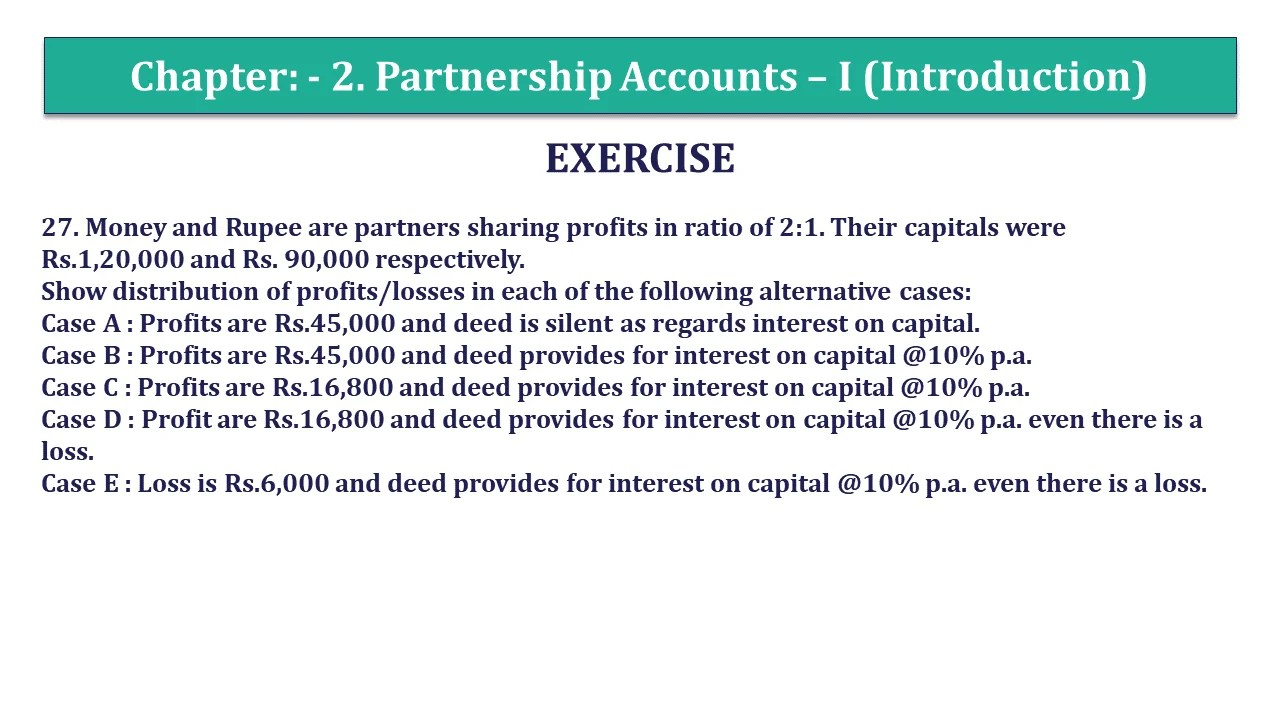# Question 27 Chapter 2 of +2 Part-1 – USHA Publication 12 Class Part – 1Q-27 - CH-2 - Usha +2 Book 2018 - Solution

Question 27 Chapter 2 of +2-Part-1

27. Money and Rupee are partners sharing profits in the ratio of 2:1. Their capitals were Rs.1,20,000 and Rs. 90,000 respectively.
Show the distribution of profits/losses in each of the following alternative cases:
Case A: Profits are Rs.45,000 and the deed is silent as regards interest on capital.
Case B: Profits are Rs.45,000 and the deed provides for interest on capital @10% p.a.
Case C: Profits are Rs.16,800 and the deed provides for interest on capital @10% p.a.
Case D: Profit is Rs.16,800 and the deed provides for interest on capital @10% p.a. even if there is a loss.
Case E: Loss is Rs.6,000 and the deed provides for interest on capital @10% p.a. even if there is a loss.

## The solution of Question 27 Chapter 2 of +2 Part-1: –

Case – A :

Money’s Share in profit 2/3 i.e. Rs.30,000
Rupee’s Share in profit 1/3 i.e. Rs.15,000
Note: No interest on capital will be allowed.

Case – B :

Interest on Money’s Capital = 1,20,000 X 10%

= Rs.12,000

Interest on Rupee’s Capital = 90,000 X 10%

= Rs.9,000

Net profit after interest on Capital:
= Rs.(45,000-12,000-9,000)

= Rs.24,000
Money’s Share in profit         = 24,000 X 2/3
= Rs.16,000
Rupee’s Share in profit         = 24,000 X 1/3
= Rs. 8000
Case – C :

Calculation of Interest on Capital

Interest on Money’s Capital = 1,20,000 X 10/100

= Rs.12,000

Interest on Rupee’s Capital = 90,000 X 10/100

= Rs.9,000

Calculation of Net Profit/Loss after Interest on Capital

Net Profit/Loss after interest on Capital = 16,800 – (12,000 + 9,000)

Net Loss = Rs. – 4,200

Calculation of Net Interest on Capital will be due to the partners after adjustment of loss:

To calculate the net amount of interest on capital payable we have to adjust the loss in capital ratio, So the loss will be Adjusted in the capital ratio of the partners:

Capital ratio of the partners = 1,20,000 :90,000

= 4:3

Interest on Money’s Capital = 12,000 – 2,400 (4200 X 4/7)

= Rs.9,600

Interest on Rupee’s Capital = 9,000 – 1,800 (4200 X 3/7)

= Rs.7,200

Case – D :

 Particulars Details Amount Interest on Capital: -Money 12,000 -Rupee 9,000 21,000 Less: Net Profit 16,800 Net Loss 4,200

Money’s Share of loss =2/3*4,200 i.e. Rs.2,800
Rupee’s Share in loss= 1/3*4,200 i.e. Rs.1,400

Case – E :

 Particulars Details Amount Interest on Capital: -Money 12,000 -Rupee 9,000 21,000 Less: Net Profit 6,000 Net Loss 27,000

Money’s Share of loss =2/3*27,000 i.e. Rs.18,000
Rupee’s Share in loss= 1/3*27,000 i.e. Rs.9,000

Comment if you have any questions.

Also, Check out the solved question of previous Chapters: –